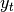# Loss Functions¶

This part of the package provides a description and mathematical background of the implemented loss functions. Every loss function can be supplied to salsa subroutines either directly (see salsa()) or passed within SALSAModel. In the definitions below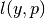stands for the loss loss function evaluated at the true label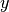and a prediction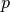.

HINGE()

Defines an implementation of the Hinge Loss function, i.e.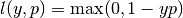.

LOGISTIC()

Defines an implementation of the Logistic Loss function, i.e.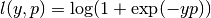.

LEAST_SQUARES()

Defines an implementation of the Least Squares Loss function, i.e.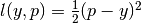.

SQUARED_HINGE()

Defines an implementation of the Squared Hinge Loss function, i.e.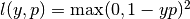.

PINBALL()

Defines an implementation of the Pinball (Quantile) Loss function, i.e.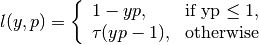If PINBALL loss is selected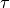parameter will be tuned by the build-in cross-validation routines.

MODIFIED_HUBER()

Defines an implementation of the Modified Huber Loss function, i.e.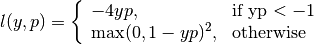loss_derivative(type)

Defines a derivative of the loss function. One can pass any type of the loss function, e.g. HINGE or an entire algorithm, for instance RK_MEANS().

Parameters: type – type of the loss function, e.g. HINGE or an entire algorithm Function which calculates a derivative at the current iterate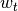, subsample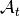and label